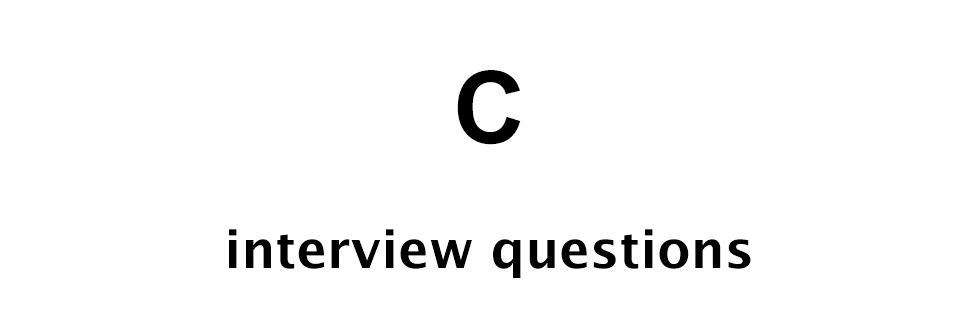# C Interview Questions - Set 1

C Programming Interview Questions## Q1: Who created C?

Dennis Ritchie is the creator of the C programming language. Brian Kernighan also contributed in the development of the language.

## Q2: Using for loop print the following pattern in C programming language

Pattern:

``````*
1 2
* * *
1 2 3 4
* * * * *
``````

For this we can write the following C code.

``````int r, c, rLim;
rLim = 5;
for (r = 0; r < rLim; r++) {
for (c = 1; c <= r + 1; c++) {
if (r % 2 == 0) {
printf("* ");
} else {
printf("%d ", c);
}
}
printf("\n");
}
``````

So, for the even rows we are printing * and for the odd rows we are printing numbers.

Click here to check out the for loop tutorial in C programming.

## Q3: What are static variables in C programming?

Static variable are the variables that retain their value.

If we have a static variable inside a function then it will retain its value between function calls.

## Q4: What is the default value for a static variable in C?

The default value for a static variable is 0.

## Q5: Write the output of the following C program

Program:

``````#include <stdio.h>

void printNumber();

int main(void) {
printNumber();
printNumber();
printNumber();

return 0;
}

void printNumber() {
static int n;
printf("n = %d\n", n);
n++;
}
``````

Following is the output of the above code.

``````n = 0
n = 1
n = 2
``````

Since, n is a static variable so the default value assigned to it is 0.

We are making 3 function calls to `printNumber()` function.

During the 1st call n is 0. Then we increment its value by 1 using the increment operator `++`. So, the value becomes 1.

In the 2nd call n is 1 then its value is incremented by 1.

In the 3rd call n is 2 and its value is incremented by 1 so, n becomes 3.

Since, we are making 3 calls so we get 0, 1 and 2.

## Q6: Write the output of the following C program

C program:

``````int i = 10;
while (i) {
printf("i = %d\n", i++);
i -= 3;
}
``````

The above code will give us the following output.

``````i = 10
i = 8
i = 6
i = 4
i = 2
``````

We are starting with i = 10. Then we are entering the while loop.

Inside the loop we are printing the value of i and in the printf statement we are incrementing the value of i by 1 using the increment operator.

Note! The value is first used then incremented.

So, i = 10 is printed then i is incremented by 1 and it becomes 11.

After printf the value of i is decreased by 3. So, i becomes 8.

So, for the next loop the value of i is 8. And this continues till value of i is not equal to 0.

When i becomes 0 we exit the loop.

## Q7: Write C program to print the ASCII code of the message "Hello World"

C code:

``````#include <stdio.h>

int main(void) {
char message[] = "Hello World";
int i;
for (i = 0; message[i] != '\0'; i++) {
printf("ASCII code of %c = %d\n", message[i], message[i]);
}
}
``````

## Q8: What will be the output of the following C program?

C program:

``````int x = 10;
int *p = &x;
*p = 20;
printf("x = %d", x);
``````

The above code will print `x = 20`.

In the above code x is assigned an interger value 10. Then its address is saved in the pointer variable p.

Next, the value of x is set to 20 via the pointer. And finally, the new value of x is printed out.

## Q9: Write C code to print the first 10 numbers of the Fibonacci series

C program to print first 10 numbers of the Fibonacci series.

``````#include <stdio.h>

int main(){
int count = 10;
int i;
int prev = 1;
int curr = 1;
int next;
printf ("%d %d ", prev, curr);
for (i = 3; i <= count; i++) {
next = prev + curr;
printf("%d ", next);
prev = curr;
curr = next;
}
printf("\n");
return 0;
}
``````

Output:

``````1 1 2 3 5 8 13 21 34 55
``````

## Q10: Write C code to check if an entered word is a Palindrome

C code:

``````#include <stdio.h>
#include <string.h>

int main(){
char str;
int beginIdx, endIdx;
int isPalindrome = 1;

//input
printf("Enter string [max 99 characters]: ");
scanf("%s", str);

for (beginIdx = 0, endIdx = strlen(str) - 1; beginIdx < endIdx; beginIdx++, endIdx--) {
if (str[beginIdx] != str[endIdx]) {
isPalindrome = 0;
break;
}
}

if (isPalindrome) {
printf("%s is a Palindrome word.\n", str);
} else {
printf("%s is not a Palindrome word.\n", str);
}

return 0;
}
``````